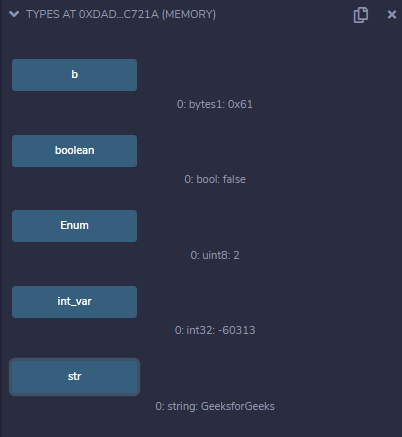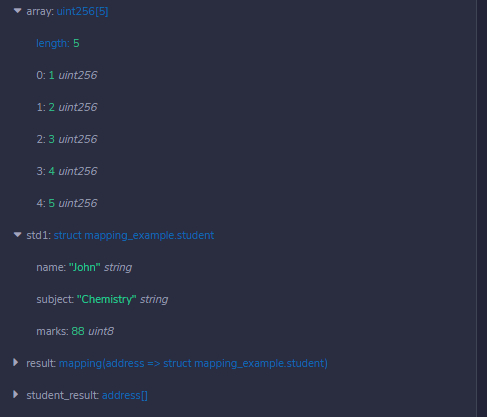# Solidity – Types

• Last Updated : 11 May, 2022

Solidity is a statically typed language, which implies that the type of each of the variables should be specified. Data types allow the compiler to check the correct usage of the variables. The declared types have some default values called Zero-State, for example for bool the default value is False. Likewise other statically typed languages Solidity has Value types and Reference types which are defined below:

### Value Types

Value type variables store their own data. These are the basic data types provided by solidity. These types of variables are always passed by value. The variables are copied wherever they are used in function arguments or assignment. Value type data types in solidity are listed below:

• Boolean: This data type accepts only two values True or False.
• Integer: This data type is used to store integer values, int and uint are used to declare signed and unsigned integers respectively.
• Fixed Point Numbers: These data types are not fully supported in solidity yet, as per the Solidity documentation. They can be declared as fixed and unfixed for signed and unsigned fixed-point numbers of varying sizes respectively.
• Address: Address hold a 20-byte value which represents the size of an  Ethereum address. An address can be used to get balance or to transfer a balance by balance and transfer method respectively.
• Bytes and Strings: Bytes are used to store a fixed-sized character set while the string is used to store the character set equal to or more than a byte. The length of bytes is from 1 to 32, while the string has a dynamic length. Byte has an advantage that it uses less gas, so better to use when we know the length of data.
• Enums: It is used to create user-defined data types, used to assign a name to an integral constant which makes the contract more readable, maintainable, and less prone to errors. Options of enums can be represented by unsigned integer values starting from 0.

Example: In the below example, the contract Types initializes the values of different types of Values Types.

## Solidity

 `// Solidity program to demonstrate` `// value types` `pragma solidity ^ 0.5.0;    ` `  `  `// Creating a contract` `contract` `Types {    `   `    ``// Initializing Bool variable ` `    ``bool` `public` `boolean = ``false``; ` `   `  `    ``// Initializing Integer variable` `    ``int32 ``public` `int_var = -60313;`   `    ``//  Initializing String variable ` `    ``string ``public` `str = ``"GeeksforGeeks"``; `   `    ``// Initializing Byte variable` `    ``bytes1 ``public` `b = ``"a"``; ` `    `  `    ``// Defining an enumerator` `    ``enum` `my_enum { geeks_, _for, _geeks }  `   `    ``// Defining a function to return ` `    ``// values stored in an enumerator` `    ``function Enum() ``public` `pure returns(` `      ``my_enum) {    ` `        ``return` `my_enum._geeks;    ` `    ``}    ` `}`

Output :### Reference Types

Reference type variables store the location of the data. They don’t share the data directly. With the help of reference type, two different variables can refer to the same location where any change in one variable can affect the other one. Reference type in solidity are listed below:

• Arrays: An array is a group of variables of the same data type in which variable has a particular location known as an index. By using the index location, the desired variable can be accessed. The array size can be fix or dynamic.
• Struct: Solidity allows users to create and define their own type in the form of structures. The structure is a group of different types even though it’s not possible to contain a member of its own type. The structure is a reference type variable which can contain both value type and reference type
• Mapping: Mapping is a most used reference type, that stores the data in a key-value pair where a key can be any value types. It is like a hash table or dictionary as in any other programming language, where data can be retrieved by key.

Example: In the below example, the contract Types initializes the values of various Reference Types.

## Solidity

 `// Solidity program to demonstrate` `// Reference Types` `pragma solidity ^0.4.18; ` ` `  `// Creating a contract` `contract` `mapping_example {`   `    ``// Defining an array    ` `    ``uint ``public` `array ` `      ``= [uint(1), 2, 3, 4, 5] ;` `    `  `    ``// Defining a Structure` `    ``struct` `student {` `        ``string name;` `        ``string subject;` `        ``uint8 marks;` `    ``}`   `    ``// Creating a structure object ` `    ``student ``public` `std1;`   `    ``// Defining a function to return ` `    ``// values of the elements of the structure` `    ``function structure() ``public` `view returns(` `      ``string memory, string memory, uint){` `        ``std1.name = ``"John"``;` `        ``std1.subject = ``"Chemistry"``;` `        ``std1.marks = 88;` `        ``return` `(` `          ``std1.name, std1.subject, std1.marks);` `    ``}` `    `  `    ``// Creating a mapping` `    ``mapping (address => student) result;` `    ``address[] student_result;` `}`

Output :My Personal Notes arrow_drop_up
Recommended Articles
Page :